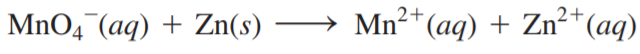×
Get Full Access to Chemistry: A Molecular Approach - 3 Edition - Chapter 18 - Problem 103e
Get Full Access to Chemistry: A Molecular Approach - 3 Edition - Chapter 18 - Problem 103e

×

# Consider the unbalanced redox reaction: Balance theISBN: 9780321809247 1

## Solution for problem 103E Chapter 18

Chemistry: A Molecular Approach | 3rd Edition

• Textbook Solutions
• 2901 Step-by-step solutions solved by professors and subject experts
• Get 24/7 help from StudySoup virtual teaching assistantsChemistry: A Molecular Approach | 3rd Edition

4 5 1 386 Reviews
15
1
Problem 103E

Consider the unbalanced redox reaction:Balance the equation and determine the volume of a 0.500 M KMnO4 solution required to completely react with 2.85 g of Zn.

Step-by-Step Solution:
Step 1 of 3

Step 2 of 3

Step 3 of 3

##### ISBN: 9780321809247

Chemistry: A Molecular Approach was written by and is associated to the ISBN: 9780321809247. This textbook survival guide was created for the textbook: Chemistry: A Molecular Approach, edition: 3. Since the solution to 103E from 18 chapter was answered, more than 281 students have viewed the full step-by-step answer. The answer to “?Consider the unbalanced redox reaction: Balance the equation and determine the volume of a 0.500 M KMnO4 solution required to completely react with 2.85 g of Zn.” is broken down into a number of easy to follow steps, and 27 words. The full step-by-step solution to problem: 103E from chapter: 18 was answered by , our top Chemistry solution expert on 02/22/17, 04:35PM. This full solution covers the following key subjects: balance, completely, consider, determine, equation. This expansive textbook survival guide covers 82 chapters, and 9454 solutions.

Unlock Textbook Solution